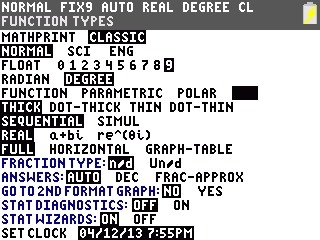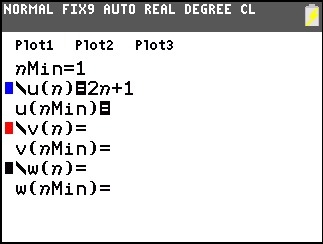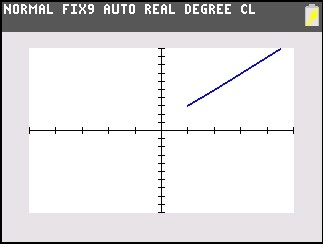# Knowledge Base

## Solution 34777: Graphing a Non-Recursive Sequence on the TI-83 Plus and TI-84 Plus Family of Graphing Calculators.

### How can I graph a non-recursive sequence on the TI-83 Plus and TI-84 Plus family of graphing calculators?

The example below shows how to graph a non-recursive sequence. A non-recursive sequence is a sequence in which the nth term is a function of the independent variable n and each term is independent of all the other terms.

NOTE: For this example, the handheld's mode settings will need to be changed.

• Press [MODE].
• Scroll down and over to SEQ and press [ENTER] to change to SEQ mode.Example: Graph the sequence function u(n)=2n+1.

• Press [Y=] to access the Y= editor.
• Press the up arrow key then press  [ENTER] to set the nMin value equal to 1.

NOTE: nMin is smallest value of n the sequence will evaluate. To input the n variable, press [X,T,q,n].

• Press  [X,T,q,N] [+]  to set u(n) equal to 2n+1.
• For the u(nMin), leave it blank.
• For the u(nMin), leave it blank.• Press [GRAPH] to display the graph.Please see the TI-83 Plus and TI-84 Plus Family guidebooks for additional information.

Last updated: 6/19/2023